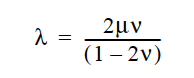# Lame LAMBDA#

Lame LAMBDA = {model_name} {float_list} [M/Lt2]


## Description / Usage#

This required card is used to specify the model for the Lame coefficient λ for the solid constitutive equation (see Sackinger, et. al., 1995). When using a nonlinear constitutive equation for ALE mesh motion, this coefficient is related to the bulk modulus:

Definitions of the input parameters are as follows:

 {model_name} Name of the Lame LAMBDA model. This parameter can have one of the following values: CONSTANT, POWER_LAW, EXPONENTIAL or USER. {float_list} One or more floating point numbers ( through ) whose values are determined by the selection for {model_name}. These are identified in the discussion of each model below.

The models are described here.

 CONSTANT For the CONSTANT model, {float_list} is a single value (see Lame MU card for relationship to other more common elastic constants): - Standard value of the elastic constant $$\lambda$$ POISSON_RATIO For any Lame MU model (see Lame MU card) this option uses the following formula to compute Lame Lame LAMBDA: - Poisson’s ratio nu.POWER_LAW The POWER_LAW model can be used in deformable porous media where the Lame coefficient varies as a power of the porosity, $$\phi$$ (Scherer, 1992):The {float_list} contains three values for this model, where: - $$\lambda_0$$ is the base Lame LAMBDA modulus at the initial porosity. - $$\phi_0$$ is the porosity in the stress-free state - m is the powerlaw exponent, as shown USER ,…, For the USER model, {float_list} is of arbitrary length, and the values are used through the param[] array in usr_lame_lambda function to parameterize a userdefined model. See examples in user_mp.c.

## Examples#

Following is a sample card:

Lame LAMBDA = CONSTANT 1.


## Technical Discussion#

Please see the Solid Constitutive Equation card for details on the use of this parameter. Special consideration is required for INCOMP* type constitutive equations. The isotropic stress term, or pressure, in that case is added onto the constitutive equation, and so this parameter must be set to zero so as to prevent any compressibility.

Important note that when one desires an incompressible solid through the use of INCOMP_PSTRAIN type models, by using an incompressible continuity equation in a LAGRANGIAN mesh region (see EQ = continuity), then the bulk modulus, or Lame Lambda expansion term is also added on. So to get a truly incompressible response, one must set the Lame LAMBDA coefficient to zero.